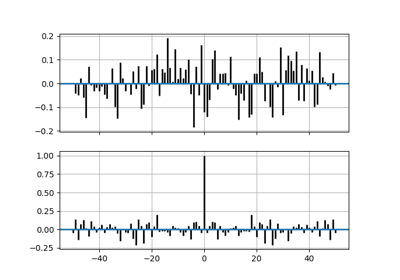/Matplotlib 3.1

# matplotlib.axes.Axes.acorr

`Axes.acorr(self, x, *, data=None, **kwargs)` [source]

Plot the autocorrelation of x.

Parameters: `x : array-like` `detrend : callable, optional, default: mlab.detrend_none` x is detrended by the detrend callable. This must be a function `x = detrend(x)` accepting and returning an `numpy.array`. Default is no normalization. `normed : bool, optional, default: True` If `True`, input vectors are normalised to unit length. `usevlines : bool, optional, default: True` Determines the plot style. If `True`, vertical lines are plotted from 0 to the acorr value using `Axes.vlines`. Additionally, a horizontal line is plotted at y=0 using `Axes.axhline`. If `False`, markers are plotted at the acorr values using `Axes.plot`. `maxlags : int, optional, default: 10` Number of lags to show. If `None`, will return all `2 * len(x) - 1` lags. `lags : array (length 2*maxlags+1)` The lag vector. `c : array (length 2*maxlags+1)` The auto correlation vector. `line : LineCollection or Line2D` `Artist` added to the axes of the correlation: `LineCollection` if usevlines is True. `Line2D` if usevlines is False. `b : Line2D or None` Horizontal line at 0 if usevlines is True None usevlines is False. `linestyle : Line2D property, optional` The linestyle for plotting the data points. Only used if usevlines is `False`. `marker : str, optional, default: 'o'` The marker for plotting the data points. Only used if usevlines is `False`.

#### Notes

The cross correlation is performed with `numpy.correlate()` with `mode = "full"`.

Note

In addition to the above described arguments, this function can take a data keyword argument. If such a data argument is given, the following arguments are replaced by data[<arg>]:

• All arguments with the following names: 'x'.

Objects passed as data must support item access (`data[<arg>]`) and membership test (`<arg> in data`).

## Examples using `matplotlib.axes.Axes.acorr`Cross- and Auto-Correlation Demo